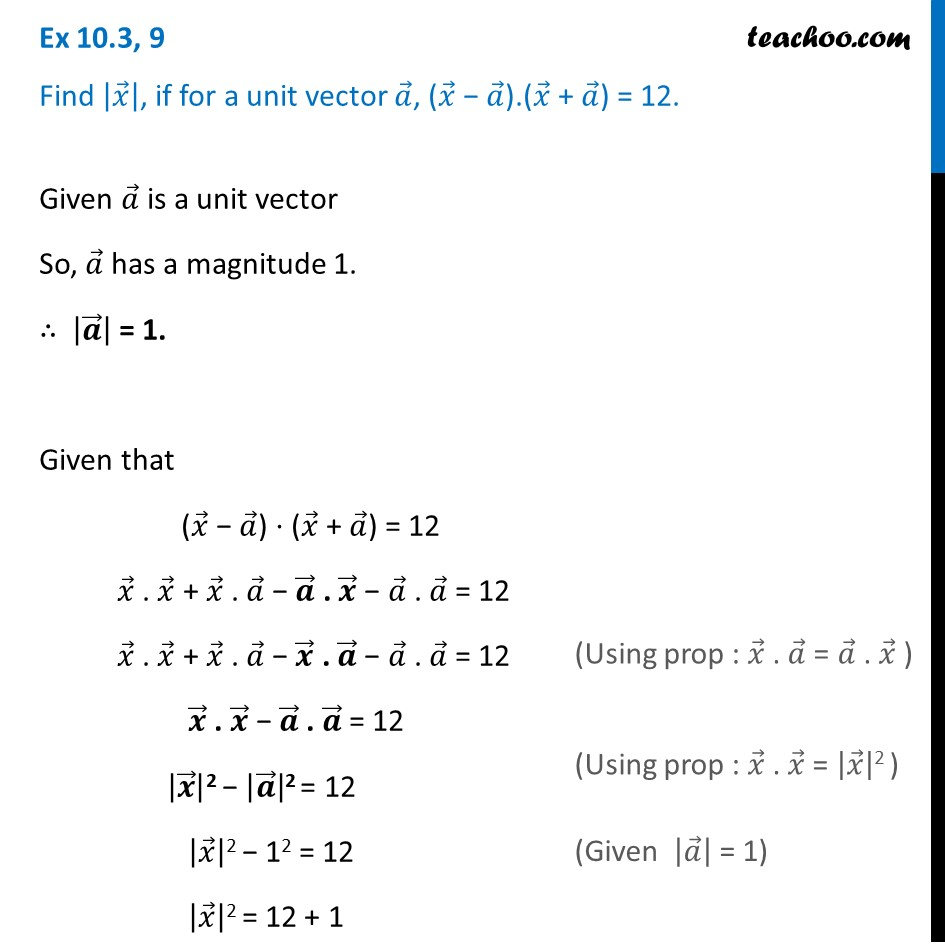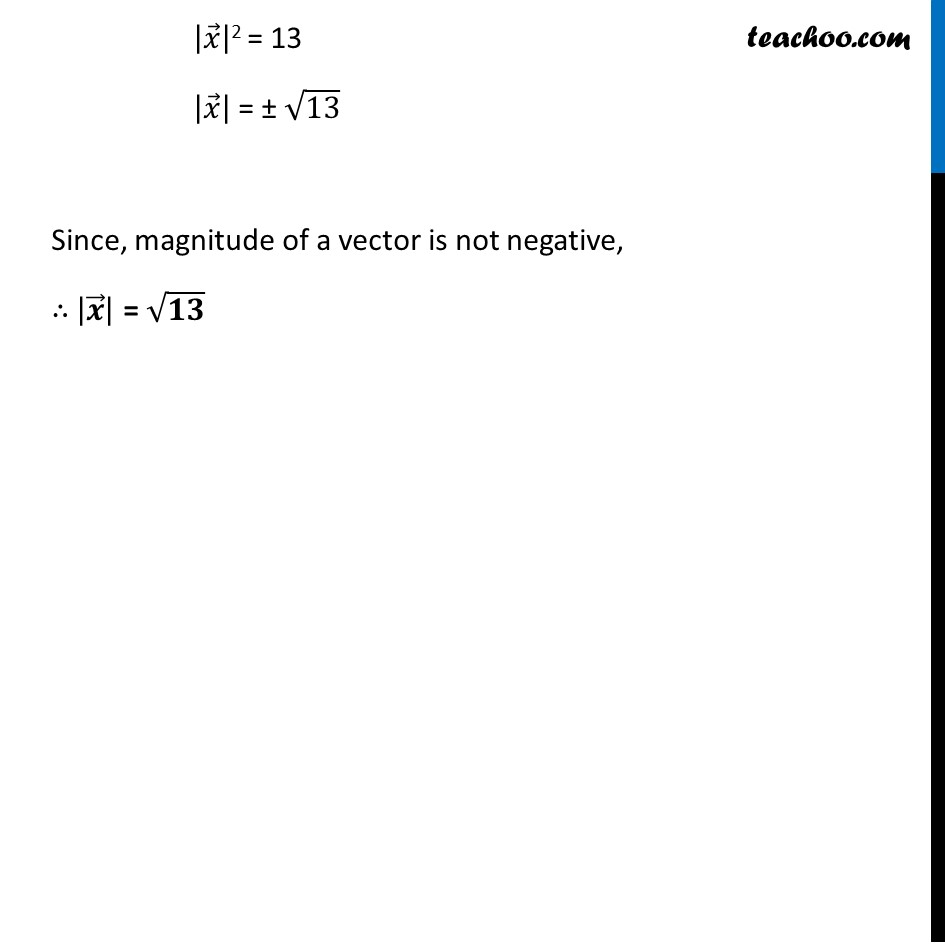Scalar product - Solving

Chapter 10 Class 12 Vector Algebra
Concept wiseLearn in your speed, with individual attention - Teachoo Maths 1-on-1 Class

### Transcript

Ex 10.3, 9 Find |𝑥 ⃗ |, if for a unit vector 𝑎 ⃗, (𝑥 ⃗ − 𝑎 ⃗).(𝑥 ⃗ + 𝑎 ⃗) = 12.Given 𝑎 ⃗ is a unit vector So, 𝑎 ⃗ has a magnitude 1. ∴ |𝒂 ⃗ | = 1. Given that (𝑥 ⃗ − 𝑎 ⃗) ⋅ (𝑥 ⃗ + 𝑎 ⃗) = 12 𝑥 ⃗ . 𝑥 ⃗ + 𝑥 ⃗ . 𝑎 ⃗ − 𝒂 ⃗ . 𝒙 ⃗ − 𝑎 ⃗ . 𝑎 ⃗ = 12 𝑥 ⃗ . 𝑥 ⃗ + 𝑥 ⃗ . 𝑎 ⃗ − 𝒙 ⃗ . 𝒂 ⃗ − 𝑎 ⃗ . 𝑎 ⃗ = 12 𝒙 ⃗ . 𝒙 ⃗ − 𝒂 ⃗ . 𝒂 ⃗ = 12 |𝒙 ⃗ |2 − |𝒂 ⃗ |2 = 12 |𝑥 ⃗ |2 − 12 = 12 |𝑥 ⃗ |2 = 12 + 1 (Using prop : 𝑥 ⃗ . 𝑎 ⃗ = 𝑎 ⃗ . 𝑥 ⃗ ) |𝑥 ⃗ |2 = 13 |𝑥 ⃗ | = ± √13 Since, magnitude of a vector is not negative, ∴ |𝒙 ⃗ | = √𝟏𝟑